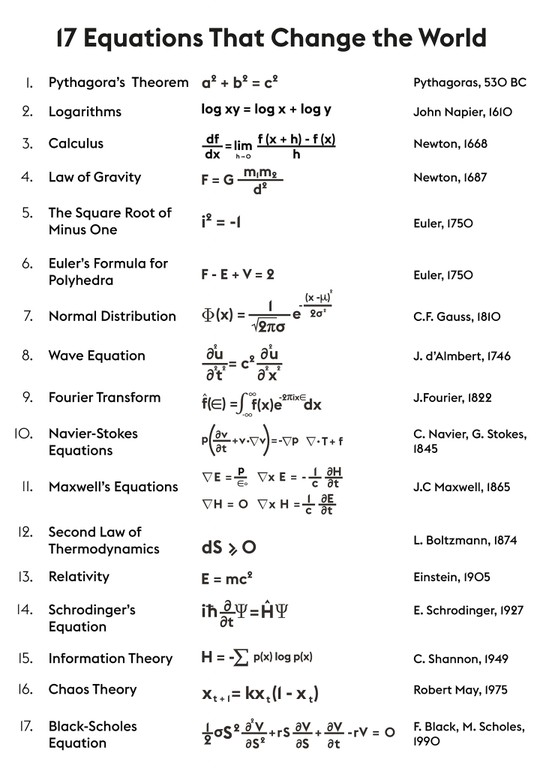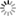Personal tools
You are here: Home Foundations of Mathematics

Foundations of Mathematics

- Overview

Through the use of abstraction and logical reasoning, mathematics developed from counting, calculation, measurement, and the systematic study of the shapes and motions of physical objects. Practical mathematics has been a human activity for as far back as written records exist. Rigorous arguments first appeared in Greek mathematics, most notably in Euclid’s Elements.

Mathematics developed at a relatively slow pace until the Renaissance, when mathematical innovations interacting with new scientific discoveries led to a rapid increase in the rate of mathematical discovery that continues to the present day.

- Foundation of Methematics

Foundations of mathematics is the study of the philosophical and logical and/or algorithmic basis of mathematics, or, in a broader sense, the mathematical investigation of what underlies the philosophical theories concerning the nature of mathematics. In this latter sense, the distinction between foundations of mathematics and philosophy of mathematics turns out to be quite vague.

Foundations of mathematics can be conceived as the study of the basic mathematical concepts (set, function, geometrical figure, number, etc.) and how they form hierarchies of more complex structures and concepts, especially the fundamentally important structures that form the language of mathematics (formulas, theories and their models giving a meaning to formulas, definitions, proofs, algorithms, etc.) also called metamathematical concepts, with an eye to the philosophical aspects and the unity of mathematics. The search for foundations of mathematics is a central question of the philosophy of mathematics; the abstract nature of mathematical objects presents special philosophical challenges.[The 17 equations that changed the world]

- Mathematics is the Mother of All Sciences

Mathematics (from Greek μάθημα máthēma “knowledge, study, learning”) is the study of quantity, structure, space, and change. Mathematicians seek out patterns and formulate new conjectures. Mathematicians resolve the truth or falsity of conjectures by mathematical proof. The research required to solve mathematical problems can take years or even centuries of sustained inquiry.

Since the pioneering work of Giuseppe Peano (1858-1932), David Hilbert (1862-1943), and others on axiomatic systems in the late 19th century, it has become customary to view mathematical research as establishing truth by rigorous deduction from appropriately chosen axioms and definitions. When those mathematical structures are good models of real phenomena, then mathematical reasoning often provides insight or predictions.

Mathematics is used throughout the world as an essential tool in many fields, including natural science, engineering, medicine, and the social sciences. Applied mathematics, the branch of mathematics concerned with application of mathematical knowledge to other fields, inspires and makes use of new mathematical discoveries and sometimes leads to the development of entirely new mathematical disciplines, such as statistics and game theory. Mathematicians also engage in pure mathematics, or mathematics for its own sake, without having any application in mind. There is no clear line separating pure and applied mathematics, and practical applications for what began as pure mathematics are often discovered.

- The Goals

The foundations of mathematics as a whole does not aim to contain the foundations of every mathematical topic. Generally, the foundations of a field of study refers to a more-or-less systematic analysis of its most basic or fundamental concepts, its conceptual unity and its natural ordering or hierarchy of concepts, which may help to connect it with the rest of human knowledge. The development, emergence, and clarification of the foundations can come late in the history of a field, and might not be viewed by everyone as its most interesting part.

Mathematics always played a special role in scientific thought, serving since ancient times as a model of truth and rigor for rational inquiry, and giving tools or even a foundation for other sciences (especially physics). Mathematics' many developments towards higher abstractions in the 19th century brought new challenges and paradoxes, urging for a deeper and more systematic examination of the nature and criteria of mathematical truth, as well as a unification of the diverse branches of mathematics into a coherent whole.

[More to come ...]

Document Actions